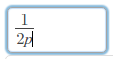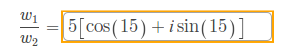How can we help?

# Why did I get the problem wrong when entering the correct math expression?

Updated

When working on math exercises that involve entering an expression, students occasionally report that they cannot enter a correct expression, even though the answer they've entered is the one shown in the hints. (We assume they'll look through the hints when an answer is unknown or marked as incorrect.)

There are three reasons this situation might arise:

1. The answer entered isn't really the same as the answer in the hints.
2. It isn't obvious how to enter the answer in the hints. The appearance of square brackets ([ ]) is the most common cause of this instance of the problem.
3. The answer in the hints is incorrect.

### First case

In the first case, for example, a hint might show that the correct answer is:When the user enters "1/2p", the answer is marked as incorrect. What's up?

The answer is in the order of operations. Remember PEMDAS? The expression as entered is equivalent to "1/2×p". So it will evaluate "1/2", then multiply the result by "p".  "1/2" times "p" is the same as "p/2". That is not the same as the correct answer specified in the hints.

To fix this, use parentheses! If you enter "1/(2p)", the evaluator has no choice but to use "2p" as the denominator, as shown in the hint. Use parentheses to show the evaluator which part of the problem to evaluate first.

### Second case

The second case involves the use of brackets. For example, a hint may display the correct answer with brackets (shown below).However, if you try to enter the answer with brackets, the problem says there are more parts to the problem. Replace the brackets with parentheses and your answer will be accepted.

### Third case

The third case is when there is an error with the hints or the question itself. Please follow the instructions in this article: Why am I getting the problem wrong when entering the correct answer?